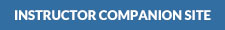McGrath:

Christian Theology: An Introduction, 6th Edition•Quizzes

•Powerpoint Slides

•Additional Materials

•Quiz Answers

•Quizzes

•Powerpoint Slides

•Additional Materials

•Quiz Answers

•Quizzes

•Powerpoint Slides

•Additional Materials

•Quiz Answers

•Quizzes

•Powerpoint Slides

•Additional Materials

•Quiz Answers

•Quizzes

•Powerpoint Slides

•Additional Materials

•Quiz Answers

•Quizzes

•Powerpoint Slides

•Additional Materials

•Quiz Answers

•Quizzes

•Powerpoint Slides

•Additional Materials

•Quiz Answers

•Quizzes

•Powerpoint Slides

•Additional Materials

•Quiz Answers

•Quizzes

•Powerpoint Slides

•Additional Materials

•Quiz Answers

•Quizzes

•Powerpoint Slides

•Additional Materials

•Quiz Answers

•Quizzes

•Powerpoint Slides

•Additional Materials

•Quiz Answers

•Quizzes

•Powerpoint Slides

•Additional Materials

•Quiz Answers

•Quizzes

•Powerpoint Slides

•Additional Materials

•Quiz Answers

•Quizzes

•Powerpoint Slides

•Additional Materials

•Quiz Answers

•Quizzes

•Powerpoint Slides

•Additional Materials

•Quiz Answers

•Quizzes

•Powerpoint Slides

•Additional Materials

•Quiz Answers

•Quizzes

•Powerpoint Slides

•Additional Materials

•Quiz Answers

•Quizzes

•Powerpoint Slides

•Additional Materials

•Quiz Answers

## Get Help With:

* These links will open a new window